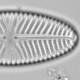## Merging taxa in assemblage data

One possible reason for the impossible percent values I’ve found in assemblages data is that taxa have been merged in Excel after percent were calculated. Doing anything in Excel is to invite disaster, if nothing else, it is very difficult to check what has been done.

Merging and renaming taxa is an almost inevitable step in the workflow for processing community and assemblages data. We need a reproducible method: here I show how can it be done with R.

I’m going to assume that the assemblage data are in wide format (one column per taxa) and that there are meta data (depths, ages etc) in one or more columns. If the meta data are in the rownames (which is very convenient for the ‘rioja’ and ‘vegan’ packages, less so for ‘dplyr’ as tibbles don’t have rownames), they can be moved into a column with `rownames_to_column`.

Here is a small artificial assemblage dataset.

```library("tidyverse")
set.seed(1)
spp <- data_frame(
depth_cm = 1:3,
sp_A = rpois(3, 5),
sp_b = rpois(3, 5),
sp.C = rpois(3, 5),
sp_D = rpois(3, 5))
spp_save <- spp # keep copy for later
spp
```
```## # A tibble: 3 x 5
## depth_cm sp_A sp_b sp.C sp_D
##
## 1 1 4 8 9 2
## 2 2 4 3 6 3
## 3 3 5 8 6 3
```

If we just want to rename a couple of taxa, the simplest solution is to use `rename`, where we set `new_name = old_name`. `rename` can take pairs of new and old names, separated by commas.

```spp %>% rename(sp_B = sp_b, sp_C = sp.C)
```
```## # A tibble: 3 x 5
## depth_cm sp_A sp_B sp_C sp_D
##
## 1 1 4 8 9 2
## 2 2 4 3 6 3
## 3 3 5 8 6 3
```

If there are many names that need altering, or we need to make the same changes to multiple data.frames, we need a different solution as rename gets tedious.

I like to make a data.frame of the old and new names and then use `plyr::mapvalues` to change the old into the new names. (`plyr` is a useful package but has several conflicts with `dplyr` so it is safer to use the `::` notation than loading it).

```changes <- read.csv(stringsAsFactors = FALSE, text =
"old, new
sp_b, sp_B
sp.C, sp_C", strip.white = TRUE)#this can go in an separate file

names(spp) <- plyr::mapvalues(names(spp), from = changes\$old, to = changes\$new)
spp
```
```## # A tibble: 3 x 5
## depth_cm sp_A sp_B sp_C sp_D
##
## 1 1 4 8 9 2
## 2 2 4 3 6 3
## 3 3 5 8 6 3
```

Merging taxa is possible in the wide format, but much easier in a thin format. We can convert from a wide format to a thin format with `gather`, and back with `spread`.

```spp <- spp_save#original version

spp_thin <- spp %>% gather(key = taxa, value = count, -depth_cm)#don't gather depth_cm
spp_thin
```
```## # A tibble: 12 x 3
## depth_cm taxa count
##
## 1 1 sp_A 4
## 2 2 sp_A 4
## 3 3 sp_A 5
## 4 1 sp_b 8
## 5 2 sp_b 3
## 6 3 sp_b 8
## 7 1 sp.C 9
## 8 2 sp.C 6
## 9 3 sp.C 6
## 10 1 sp_D 2
## 11 2 sp_D 3
## 12 3 sp_D 3
```

If there are just a few taxa that need merging, we can use `recode` within `mutate` followed by `summarise`. Note that in contrast with `rename`, `recode` expects “old_name” = “new_name”

```spp_thin %>%
mutate(taxa = recode(taxa, "sp.C" = "sp_D")) %>%
group_by(depth_cm, taxa) %>%
summarise(count = sum(count)) %>%
spread(key = taxa, value = count)
```
```## # A tibble: 3 x 4
## # Groups: depth_cm 
## depth_cm sp_A sp_b sp_D
## *
## 1 1 4 8 11
## 2 2 4 3 9
## 3 3 5 8 9
```

If there are many taxa that need merging (or some that need merging and some renaming) we can use `mapvalues` again.

```changes <- read.csv(stringsAsFactors = FALSE, text =
"old, new
sp_b, sp_B
sp.C, sp_D", strip.white = TRUE)#this can go in an separate file

spp_thin %>%
mutate(taxa = plyr::mapvalues(taxa, from = changes\$old, to = changes\$new)) %>%
group_by(depth_cm, taxa) %>%
summarise(count = sum(count)) %>%
spread(key = taxa, value = count)
```
```## # A tibble: 3 x 4
## # Groups: depth_cm 
## depth_cm sp_A sp_B sp_D
## *
## 1 1 4 8 11
## 2 2 4 3 9
## 3 3 5 8 9
```

This can also be done with a `left_join`.

```spp2 <- spp_thin %>%
left_join(changes, by = c("taxa" = "old")) %>%
mutate(taxa = coalesce(new, taxa)) %>% #takes original name if no new one.
select(-new) %>%
group_by(depth_cm, taxa) %>%
summarise(count = sum(count)) %>%
spread(key = taxa, value = count)
spp2
```
```## # A tibble: 3 x 4
## # Groups: depth_cm 
## depth_cm sp_A sp_B sp_D
## *
## 1 1 4 8 11
## 2 2 4 3 9
## 3 3 5 8 9
```

Now the data are ready for further analysis – remember some functions will want you to remove the meta_data first. For example

```cca(select(spp2, -depth_cm))
```